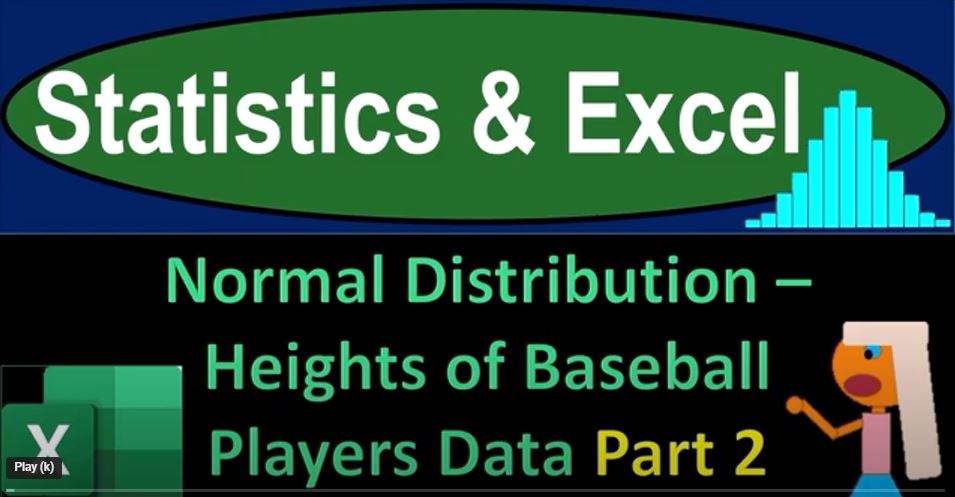## Normal Distribution – Hight’s of Baseball Players Data Part 2 1622 Statistics & Excel

Are you ready to dive into the world of statistics, Excel, and the heights of baseball players? In this blog post, we’ll take you through a step-by-step journey as we explore the heights of baseball players’ data and create insightful graphs using Microsoft Excel.

Introduction

Before we dive into the world of data analysis and graph creation, let’s set the stage. We’ll be working with data related to baseball player heights, a common dataset found in various sources. In our case, we’ll use this data to practice statistical analysis and graph plotting. If you don’t have a dataset at hand, websites like Kaggle.com offer a wealth of baseball-related data for your practice.

Part 1: Data Collection and Initial Analysis

Our journey begins with a blank Excel worksheet, and from there, we’ll build our analysis step by step. Here’s what we’ve covered so far:

1. Data Collection: We started with a blank worksheet and collected data related to baseball player heights.
2. Data Exploration: We sorted and organized the data, creating a table to extract the relevant information – the heights of the players.
3. Statistical Analysis: We calculated key statistics such as the mean, standard deviation, median, and mode. Notably, we observed that the mean, median, and mode were similar, suggesting a bell-shaped distribution, which we might approximate with a normal distribution.
4. Creating a Bell Curve: We proceeded to create a bell curve, determining the lower and upper bounds for the x-axis, which represents player heights. We used the NORM.DIST function to model the normal distribution.
5. Comparing Data with Normal Distribution: We visualized the dataset on a graph and compared it with a normal distribution. The close similarity further supported our choice to use the bell curve for prediction.

Part 2: Graphical Representation and Questions

Now, let’s move on to the next phase where we explore various questions and create graphical representations:

Dynamic Labels: We made our labels dynamic, allowing us to change parameters like the threshold (e.g., 79 inches) and immediately see the impact on our analysis. This feature will come in handy for future questions.

Calculating Z-Scores: We introduced the concept of Z-scores, which indicate how far a data point is from the mean in terms of standard deviations. Understanding Z-scores helps us assess where a data point falls within the distribution.

Creating Graphs: We plotted normal distribution graphs using the NORM.DIST function for different scenarios, such as “P(X ≥ 79)” and “P(X ≤ 79),” to visualize the areas under the curve.